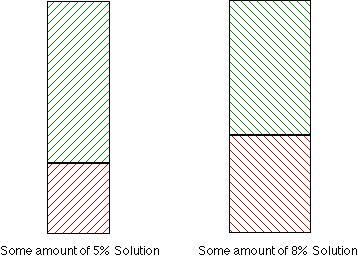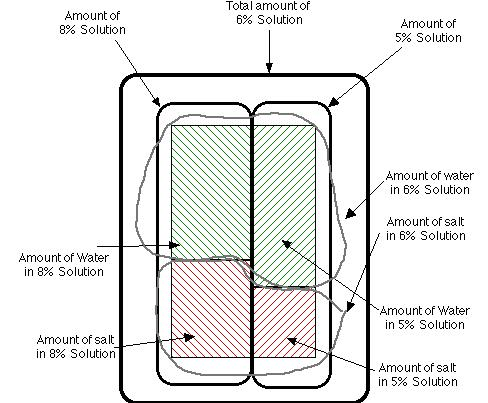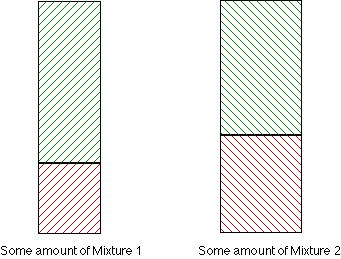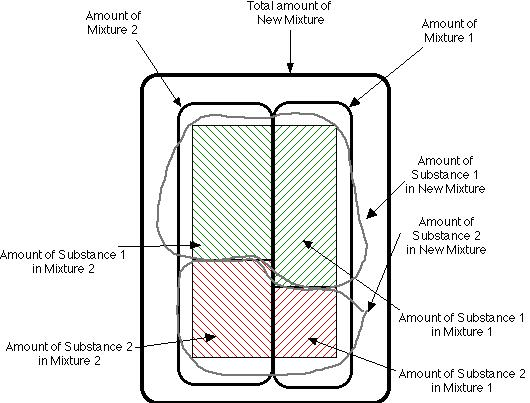# How to think about "Mixture" Problems

## Math Student Teaching Spring 2000 Pat Thompson, Vanderbilt University

 We want to make 300 g of a salt solution containing 6% salt. We will make it by pouring together two other salt solutions, one containing 8% salt and the other containing 5% salt. How many grams of the 5% solution and how many grams of the 8% solutions should we mix?

### Things to notice about the problem

• There are only two substances involved -- salt and water.
• We mix salt and water in various proportions to make solutions of different strengths. Any particular solution is named by the percent of its weight (or mass) that is salt. A solution is called "5% solution" is called that because 5% of it is salt.
• When we pour together two different solutions, we get a new solution. The new solution is made of water and salt only. All the water in the new solution came from the water in the two solutions we mixed. All the salt in the new solution came from the two solutions we mixed.
• The weight of the salt in the new solution makes some percent of the new solution's weight.

## We can organize our understanding of this problem as such:

### Start off with two mixtures:### Combine them to make a new mixture### Things we can deduce from the information in this problem

• We want to make 300 g of a salt solution containing 6% salt.
So, the amount of salt in the new solution is 6% of 300 g, or 18 grams.

• We will make it by pouring together two other salt solutions, one containing 8% salt and the other containing 5% salt.
However much 8% solution we use, 8 percent of it will be salt. If we use x grams of 8% solution, it will be made of .08x grams of salt. If we use .08x grams of salt from the 8% solution, we must get the rest of the salt from the 5% solution. So the amount of 5% solution we use will contain 18-.08x grams of salt.

• How many grams of the 5% solution and how many grams of the 8% solutions should we mix?
Any amount of 5% solution is 20 times as large as the amount of salt in it. So, if we get 18-.08x grams of salt from the 5% solution, we must use 20(18-.08x) grams of 5% solution.

We are making 300 grams of 6% solution, and that comes from combining the two amounts we start with. So, 300 = (number of grams of 8% solution) + (number of grams of 5% solution).

Therefore:
300 = x + 20(18-.08x)
300 = x + 360 - 1.6x
-60 = -.6x
100 = x. Use 100 grams of 8% solution and 20(18-8), or 200 grams of 5% solution.

# We can use a table to organize our thinking

1. We know we will have 300 grams of solution that is 6% salt.
2. 300 grams of solution that is 6% salt contains 18 grams of salt.
3. Assume we have x grams of 8% solution.
4. x grams of solution that is 8% salt will contain .08x grams of salt.
5. Since there are 18 grams of salt altogether and .08x grams of it comes from the 8% solution, the rest of it, 18-.08x grams, must come from the 5% solution.
6. For the 5% solution to be 5% salt, the entire amount of solution must weigh 20 times as much as the salt in it. Therefore, there is 20(18-.08x) grams of 5% solution.
7. The two amounts of solution must total 300 grams when combined. Therefore,
300 = x + 20(18 - .08x).# We can generalize this understanding

### Start off with two amounts of two mixtures### Combine them to make a new mixture# Use a table to organize your thinking